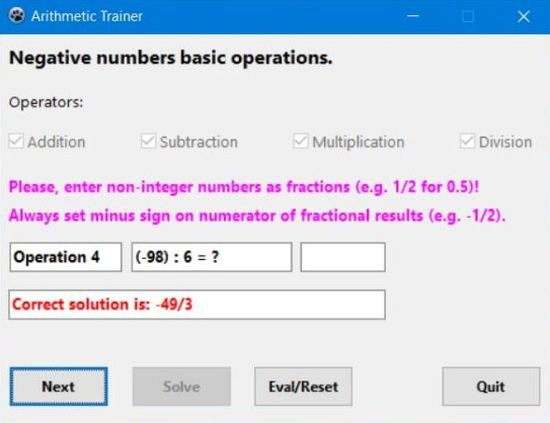# Computing: Free Pascal Programming

 Programming Home Home Contact

## Maths trainer: Negative numbers operations (Arithmetic4).

Description: Generation of exercises concerning basic arithmetic operations with negative integer numbers. The operations (addition, subtraction, multiplication and division) may be chosen by the user.

Please, note that the non integer results (of a division) have to be entered as a fraction and that this fraction must be reduced as much as possible. Thus, if the question is, for example, "25 / (-10)", the only answer accepted as correct by the program is "-5/2". Also note that if the result is a negative fraction, the minus sign has to be used with the numerator. Thus, in the example above, an answer of "5/-2" will be considerd as false!

Use the "Start" button to begin the test; after filling in the result, push "Solve" to check your answer, then "Next" for the next exercise. Pushing "Eval/Reset" shows your success percentage and re-enables the operator selection for a new test.

Free Pascal features: Basic controls: checkboxes, edit fields, (push) buttons.

Screenshot: